﻿﻿ Electromotive Force of NFC Coilexamples｜products｜Murata Software Co., Ltd.# Example34Electromotive Force of NFC Coil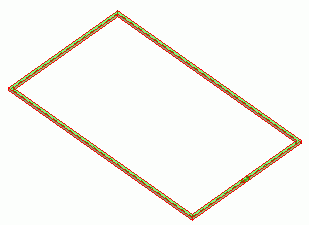### General

• An NFC coil is placed in AC magnetic field.
The electromotive force is generated by the current induced in the coil.

• Integral path is required to be set in the electric circuit.

• The electromotive force, the magnetic field vectors and the magnetic flux density are solved.

• Unless specified in the list below, the default conditions will be applied.

### Analysis Space

 Item Settings Analysis Space 3D Model unit mm

### Analysis Conditions

 Item Settings Solvers Magnetic Field Analysis [Gauss] Analysis Type Harmonic analysis Options N/A

The frequency is set to 13.56[Hz] on the harmonic analysis tab.

The external magnetic field is directing the positive Z direction.

Thus, the external magnetic field is set to be AC with the frequency of 13.56[Hz].

 Tab Setting Item Settings Mesh Tab Frequency-Dependent Meshing Reference frequency: 13.56×10^6[Hz] Harmonic analysis Sweep Type Single frequency Frequency 13.56×10^6[Hz] External magnetic field External magnetic field X=0, Y=0, Z=1[A/m]

### Model

An NFC coil (Coil) is defined.

The coil size is 72[mm] x 42[mm].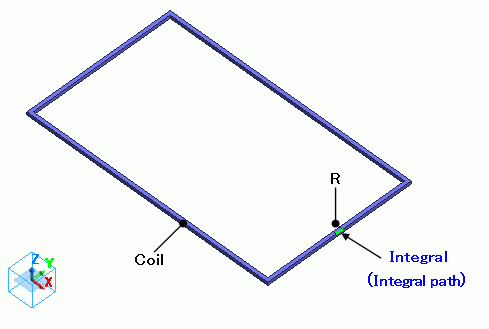Coil is terminated with a body R having sufficient resistance to measure the electromotive force.

If the boundary condition of integral path is set along the current path, the electromotive force can be calculated by integrating the electric field (current density*resistance) on the integral path.

Basically, the body R is not needed when setting the integral path along the current path of the loop coil. However, the current density is not constant at the section of the coil and calculation cannot be done accurately.

By adding the body R having resistance high enough,

the electric potential difference is mostly generated at the body R, and the current density at the section of the body R is almost constant. So the calculation will be accurate.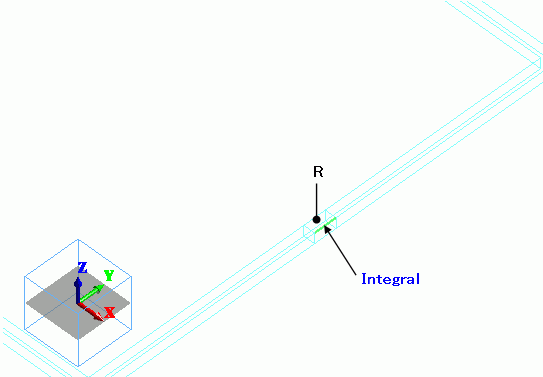### Body Attributes and Materials

 Body Number/Type Body Attribute Name Material Name 6/Solid Coil 008_Cu * 5/Solid R R

* Available from the Material DB

It is assumed that the induced current flows in Coil in this exercise.
Therefore no settings are required for the Current tab of the body attribute and I/O port boundary condition.

The electric conductivity of the body R is set low enough compared to the coil as follows.

 Material Name Tab Settings R Conductor wall Conductivity Type: Conductor Conductivity: 1[S/m]

### Boundary Conditions

Integral path is set in the current path of the body R in order to calculate the electromotive force.

The outer boundary condition is magnetic wall in this exercise.

 Boundary Condition Name/Topology Tab Boundary Condition Type Settings Integral/Face Electric Integral path Outer Boundary Condition Electric Magnetic wall

### Results

To see the electromotive force, go to the [Results] tabclick [Table]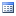.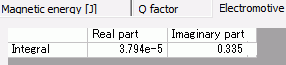The absolute value is the amplitude of the electromotive force.

It is pretty close to the theoretical value: V=jωSB=j*2*π*13.56*10^6*0.072*0.042*1*μ0=j0.324[V].

The vectors of current density are shown below.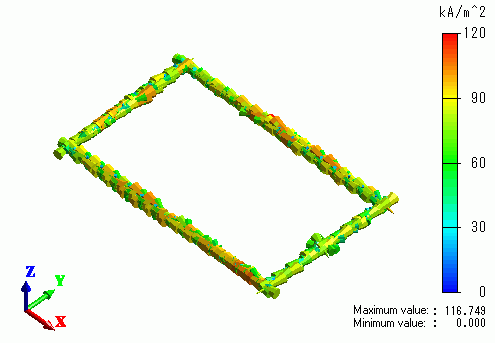The magnetic field sectioned at YZ plane is shown below.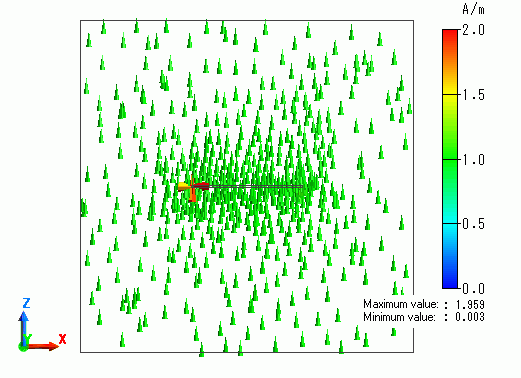The magnetic field is created as set in the direction tab.

﻿ ﻿